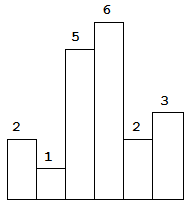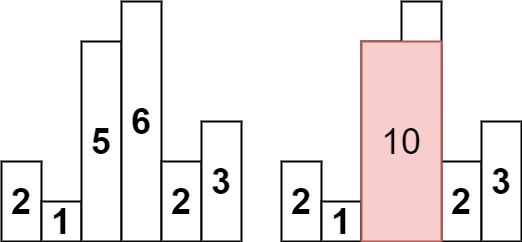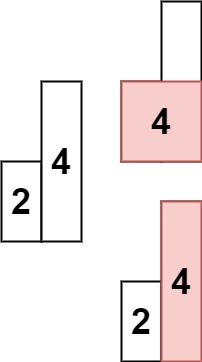84. Largest Rectangle in Histogram

Given n non-negative integers representing the histogram's bar height where the width of each bar is 1, find the area of largest rectangle in the histogram.Above is a histogram where width of each bar is 1, given height = `[2,1,5,6,2,3]`.The largest rectangle is shown in the shaded area, which has area = `10` unit.

Example:

```Input: [2,1,5,6,2,3]
Output: 10
```

Example 1:```Input: heights = [2,1,5,6,2,3]
Output: 10
Explanation: The above is a histogram where width of each bar is 1.
The largest rectangle is shown in the red area, which has an area = 10 units.
```

Example 2:```Input: heights = [2,4]
Output: 4
```

Constraints:

• `1 <= heights.length <= 105`
• `0 <= heights[i] <= 104`

84. Largest Rectangle in Histogram
``````struct Solution;

impl Solution {
fn largest_rectangle_area(mut heights: Vec<i32>) -> i32 {
let mut stack: Vec<(i32, i32)> = vec![(0, 0)];
heights.push(0);
let n = heights.len();
let mut res = 0;
for i in 0..n {
let x1 = (i + 1) as i32;
let y1 = heights[i];
let mut x3 = x1;
while let Some(&(x2, y2)) = stack.last() {
if y2 > y1 {
stack.pop();
res = res.max((x1 - x2) * y2);
x3 = x2;
} else {
stack.push((x3, y1));
break;
}
}
}
res
}
}

#[test]
fn test() {
let heights = vec![2, 1, 5, 6, 2, 3];
let res = 10;
assert_eq!(Solution::largest_rectangle_area(heights), res);
let heights = vec![2, 1, 2];
let res = 3;
assert_eq!(Solution::largest_rectangle_area(heights), res);
}
``````